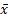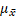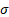# Statistics

## The Central Limit Theorem

The Central Limit Theorem states that the averages (means) of randomly selected groups (samples) of data points taken from a population have an approximately normal distribution, whether the population is normally distributed or not. This is very useful: it means we can apply the normal distribution tools to find probabilities to almost any significantly large population!

A sampling distribution is a picture of what we get when we randomly select groups of data points from our population, and calculate the means of those groups (the sample means). So, instead of having all the data points from the population plotted for a picture, we plot the means of these samples.

The more data points you have in each sample, the more normal these means are distributed. The standard size for samples when using the Central Limit Theorem is $$n\geq 30$$ though smaller samples are permitted if the population is normally distributed to begin with.

As the sample size increases, the spread of the values decreases (the distribution gets ‘narrower’ when we draw a picture). A decrease in spread means a decrease in the variance and standard deviation, so the standard deviation of the sampling distribution changes accordingly:

$\sigma_{\bar{x}} = \frac{\sigma}{\sqrt{n}}$

This information is used to rewrite the Z formula for cases when, instead of using data from a whole population, we are using data from the distribution of the sample means:

$z=\frac{\bar{x} - \mu_\bar{x}}{\frac{\sigma}{\sqrt{n}}}$= sample mean= mean of the sampling distribution (= population mean)= standard deviation of the population

n = number of data points in each sample

This video provides a review of the definition of the Central Limit Theorem:

The next three videos are solutions to parts (a), (b) and (c) of the following question:

The length of time, in hours, that it takes a group of beginner golfers to play a 9-hole game is normally distributed with a mean of 2.5 hours and a standard deviation of 24 minutes. Find the probability that the mean of 27 random samples of golfers is:

a) Less than 2.45 hours

b) Greater than 2.45 hours

c) Between 2.3 and 2.5 hours